Register for Maths, Science, English, Reasoning Olympiad Exams Register here | Book Free Demo for Live Olympiad Classes here | Check Olympiad Exam Dates here | Buy Practice Papers for IMO, IOM, HEO, IOEL etc here | Login here to participate in all India free mock test on every Saturday

# Basic Geometrical IdeasGeometry in Mathematics is very important branch of Mathematics; it includes all the shapes, size, and relative position of figures and the properties of space. Here we are going to learn about the basics of different shapes, what are their properties and more importantly about their importance.

Geometry is derived from a Greek work called “Geometron" .Geo means Earth and metron means measurement.

Points

• A pointin geometry is a location.

• It has no size i.e. no width, no length and no depth and the pointis shown by a dot.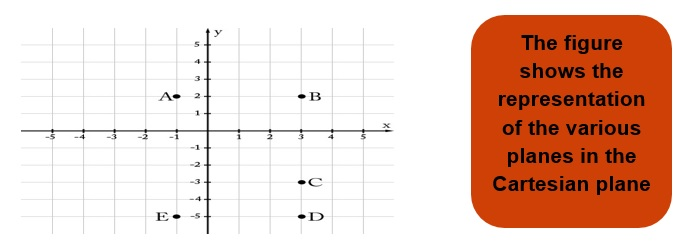• In Mathematics a lineis a straight one-dimensional figure, which has no thickness and can extend infinitely in both directions.

• Here an important point is to be remembered that a line is always straight and has no end points.

• A line segment is a type of line which has definite end points that is its length can only be infinite if the two end points are very-very far from each other.

• In a line segment all the points will lie within those end points.

• A rayis a part of a line that has one endpoint and goes on infinitely in only one direction.

Intersecting Lines: If two lines have atleast one common point then these are called as intersecting lines.

Parallel Line: If two lines never meet that is they don’t have any common point are called as parallel.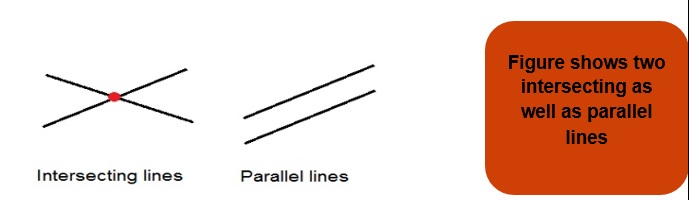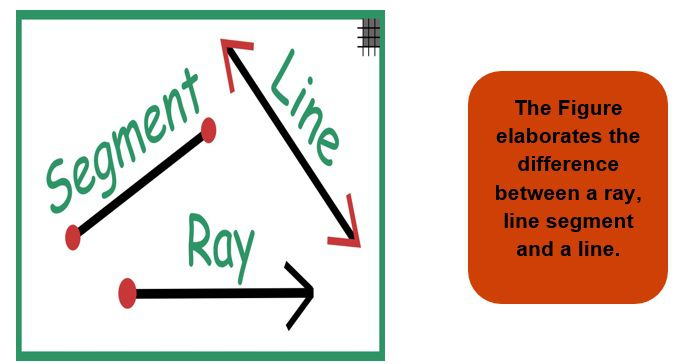Curves

In mathematics any figure which is not straight can be termed as curve, thus in simple words we can say that a curve is a line but it need not to be straight.

There are basically two types of curves

1. a) Open curve: The curve whose two ends don’t meet are called as open curve.
2. b) Closed curve: The curve whose two end points finally meet are called as closed curve.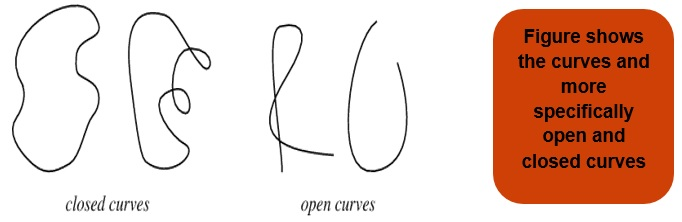Polygons

In geometry, a polygon is a plane figure made by atleast 3 line segments joined in such a manner to obtain a closed figure.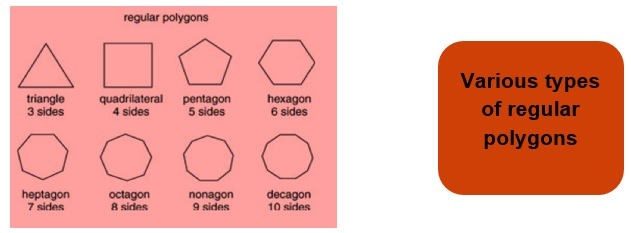Angles

• When two rays start from a common end point then an angle is made at the common end points.

• The two rays forming the angle are called the arms or sides of the angle.

• The common end point is the vertex of the angle.

While writing the name of an angle the name of the vertex is always written in the middle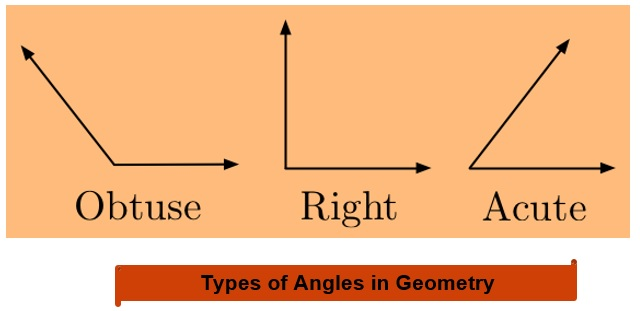Obtuse Angle: When the angle is greater than 90°.

Acute Angle: When the angle is less than 90°.

Right Angle: When the angle is exactly 90°.

Triangles

A triangle is 3 sided polygon which means it is made from three line segments which are joined in such a way to form a closed figure. And this closed figure is called as Triangle.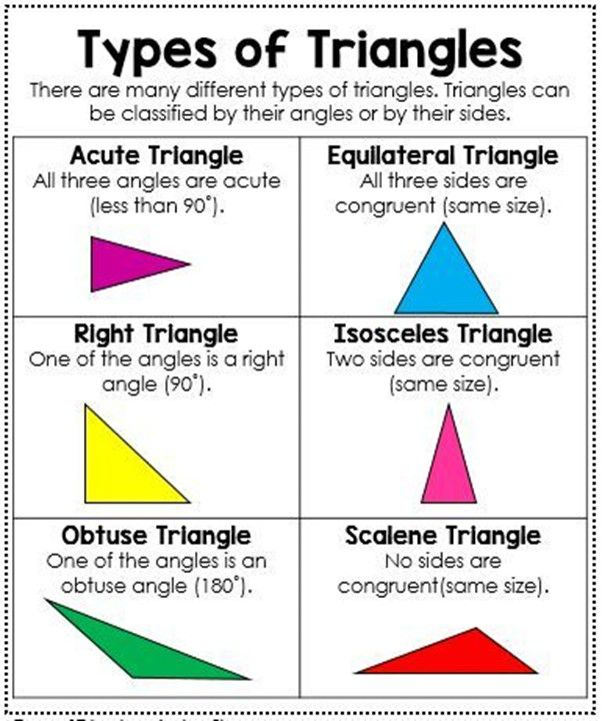A four sided polygon is called as quadrilateral. Squares, Rectangles, Parallelograms are all quadrilaterals.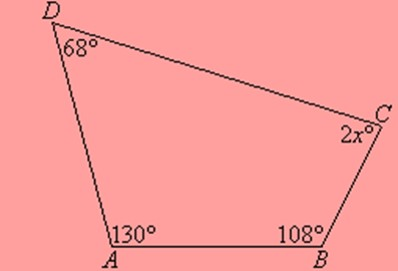Here angle BAC and angle DCB are called as opposite angles and same with angle ADC and angle ABC.

Circles

• A circle is a simple closed curve which is not a polygon.

• It is the set of all points in a plane that are at a given distance from a given point called the Centre.

• The curve traced out by a point which moves such that its distance from a fixed point called Centre is constant.

• The distance from Centre to the point is called as the radius of the circle.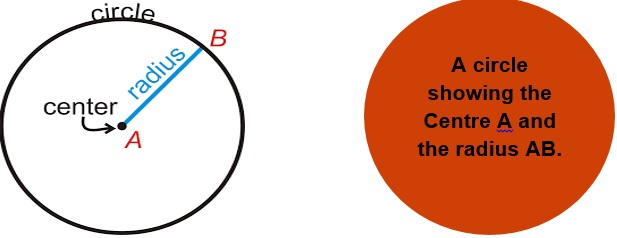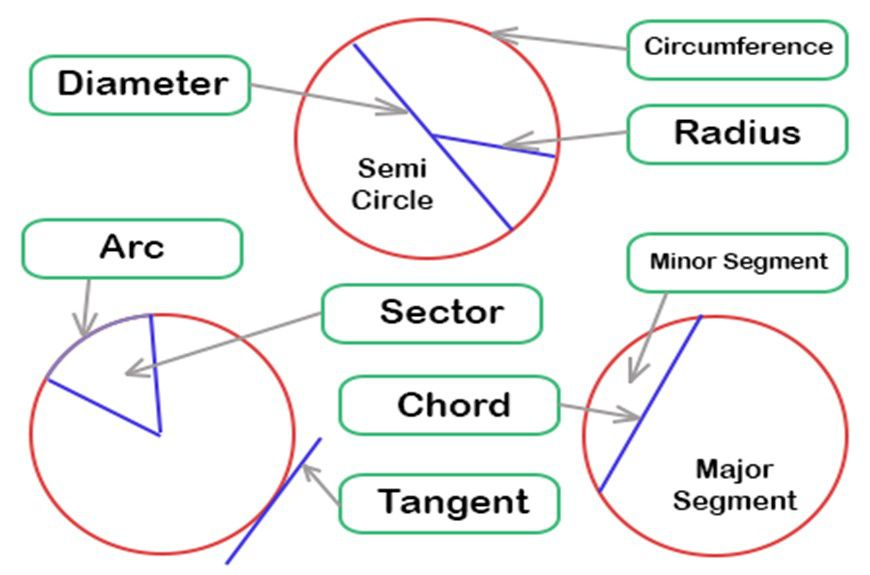• A chord of a circle is a line segment joining any two points on the circle.

• A diameter is a chord passing through the Centre of the circle.

• A sector is the region in the interior of a circle enclosed by an arc on one side and a pair of radii on the other two sides.

• A segment of a circle is a region in the interior of the circle enclosed by an arc and a chord.
• The diameter of a circle divides it into two semi-circles.

Practice Questions

Q1) Differentiate between Line, Line Segment and Ray.

Q2) How many sides are there in a triangle?

Q3) Try to find out and identify different shapes present in your class and home.

Recap

• A point determines a location.

• A line segment corresponds to the shortest distance between two points.

• Two distinct lines meeting at a point are called intersecting lines and two lines in a plane are said to be parallel if they do not meet.

• A polygon is a simple closed curve made up of line segments.

• A chord of a circle is a line segment joining any two points on the circle and diameter is a chord passing through Centre.

• A triangle is a three-sided polygon whereas a quadrilateral is a four sided polygon.

## Quiz for Basic Geometrical Ideas

 Q.1 The line that bisects an angle is known as its _________ a) Angular bisector b) Arc c) Base d) none of the above
 Q.2 To divide a line segment into 4 equal parts, how many perpendicular bisectors are needed? a) 4 b) 3 c) 2 d) 5
 Q.3 An angle of 160° is bisected using an angular bisector. What is the measure of each angle obtained? a) 100° and 60° b) 80° each c) 90° and 70° d) none of the above
 Q.4 A circle of radius 4.2 cm has a diameter equal to_________. a) 4.2 cm b) 2.1 cm c) 8.4 cm d) none of the above
 Q.5 Which of the figures are not symmetrical about its diagonal? a) Rhombus b) Square c) Both a and b d) Rectangle
 Q.6 Which of these angles can be constructed using a ruler and a compass? a) 45° b) 80° c) 100° d) All
 Q.7 How would one draw an angle of 15° using a ruler and a compass? a) 15° can be drawn directly using a ruler and a compass. b) First draw 60° using a ruler and a compass and then draw its angular bisector. Next draw the angular bisector of the previously bisected angle. c) It cannot be drawn using a ruler and compass. d) Draw a 30° and then bisect it using a ruler and a compass.
 Q.8 A line segment PQ of length 6.6 cm is bisected at O. Find the length of PO a) 6.6 cm b) 3 cm c) 3.3 cm d) 13.2 cm
 Q.9 A line segment PQ of length 6.6 cm is bisected at O. Find the relation between PO and OQ. a) PO>OQ b) PO c) PO≥OQ d) OP=OQ
 Q.10 Two lines are said to be perpendicular, if _________ a) they intersect at 90° b) they do not intersect c) they intersect at any random angle d) none of the above